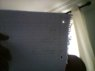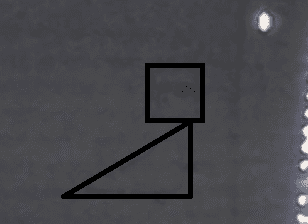# Crate on a frictionless ramp

• abelmoore
In summary, the loot crate moves down a frictionless ramp at a rate of 5m/s. The net force and acceleration are -5.8N and -9.8m/s2. After reaching the flat surface, the loot crate has a net force of -5.8N and an acceleration of -9.8m/s2.f

## Homework Statement

Consider a loot crate, at the top of a frictionless ramp. If the mass of the loot crate is 11.5kg and the ramp has a rise of 3m and a run of 4m, then compute the following.

a) Compute the free body diagram of the loot crate a time 0. (i.e. when the loot crate is at the top of the ramp.)
b) Compute the net force and the acceleration of the loot crate at time 0. Given the frictionless surface what do we know about the acceleration as the object moves down the ramp?
c) Consider the loot crate at it leaves the ramp and moves onto a flat surface that now has some friction. Compute the free body diagram for this situation. If the force of friction is proportional to
one quarter of the acceleration of the loot crate, calculate the new net force and acceleration at this point.
d) If we assume that the force of friction is constant after this point. How long will it take for the loot crate to stop moving?

## Homework Equations

not sure of formula

## The Attempt at a Solution

i drew a picture of a loot crate and a ramp need some hints

is it visible

#### Attachments

•diagram math.jpg
27.1 KB · Views: 296
Thanks for trying. It might help to put something dark - the rest of the book maybe - behind to stop the light going through. You want light reflected off the front (writing side) of the paper.That's about as much as I can see.
The block usually has a flat side lying on the slope.

Now you mark in any forces acting on the block.

#### Attachments

Mod note: Thread title changed to be more descriptive of the problem.

i did a = gsincirclethingy -9.8 sin 36.87 a = -5.8
mgsincirclethingy 11.5x-9.8sin 36.87 67
need help for c and d
As mentioned on another thread, please take the trouble to use the special characters. Leave blanks, e.g. g sin, not gsin.
There was no point in finding the angle. You are given the slope is 3m vertically and 4m horizontally, so the slope must be 5m. This gives you exact values for sin and cos.

Please do not post handwritten algebra as images. That is for textbook extracts and diagrams. Yours is utterly unreadable.